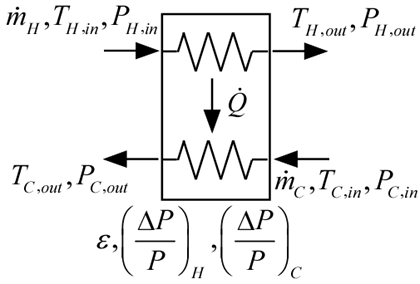Contents - index

HeatExchanger2_CLThe procedure HeatExchanger2_CL models a heat exchanger in which two fluids interact with one another.  The approach temperature difference is specified.  In this model, the pinchpoint must occur at either the hot or cold end; this model will not work if there is a chance that the pinchpoint will occur within the heat exchanger.  The procedure supports real fluids, ideal gas, incompressible, or brines on either side.  See HeatExchanger3_CL for a model that calculates the heat transfer coefficients to determine the heat exchanger performance.

Inputs:

F_H\$: hot fluid string identifier

C_H: concentration of hot fluid - only necessary when hot fluid is brine, otherwise set C_H=0

m_dot_H: mass flow rate of hot fluid (kg/s or lbm/hr)

h_H_in: inlet specific enthalpy of hot fluid (J/kg, kJ/kg, or Btu/lbm)

P_H_in: inlet pressure of hot fluid (bar, atm, Pa, kPa, MPa)

F_C\$: cold fluid string identifier

C_C: concentration of cold fluid - only necessary when cold fluid is brine, otherwise set C_C=0

m_dot_C: mass flow rate of cold fluid (kg/s or lbm/hr)

h_C_in: inlet specific enthalpy of cold fluid (J/kg, kJ/kg, or Btu/lbm)

P_C_in: inlet pressure of cold fluid (bar, atm, Pa, kPa, MPa)

DT: approach temperature difference (K, C, R, or F)

DPoverP_H: pressure drop normalized by absolute pressure on hot side (-)

DPoverP_C: pressure drop normalized by absolute pressure on cold side (-)

Outputs:

h_H_out: outlet specific enthalpy of hot fluid (J/kg, kJ/kg, or Btu/lbm)

P_H_out: outlet pressure of hot fluid (bar, atm, Pa, kPa, MPa)

h_C_out: outlet specific enthalpy of cold fluid (J/kg, kJ/kg, or Btu/lbm)

P_C_out: outlet pressure of cold fluid (bar, atm, Pa, kPa, MPa)

Q_dot: heat transfer rate from hot to cold (W, kW or Btu/hr)

eff: effectiveness (-)

Example:

\$UnitSystem SI Mass J K Pa

F_H\$='CarbonDioxide'

m_dot_H=0.1 [kg/s]

T_H_in=380 [K]

P_H_in=4e6 [Pa]

h_H_in=enthalpy(F_H\$,T=T_H_in,P=P_H_in)

m_dot_C=0.03 [kg/s]

T_C_in=300 [K]

P_C_in=6e6 [Pa]

C_C=10 [%]

h_C_in=enthalpy(F_C\$,T=T_C_in,C=C_C,P=P_C_in)

F_C\$='PG'

DT=5 [K]

DPoverP_H=0.01

DPoverP_C=0.01

Call heatexchanger2_cl(F_H\$, 0, m_dot_H, h_H_in, P_H_in, F_C\$, C_C, m_dot_C, h_C_in, &

P_C_in, DT, DPoverP_H, DPoverP_C: h_H_out, P_H_out, h_C_out, P_C_out, Q_dot, eff)

{Solution:

h_H_out = -36618 [J/kg]

P_H_out = 3.960e6 [Pa]

h_C_out = 384988 [J/kg]

P_C_out = 5.940e6 [Pa]

Q_dot = 8544 [W],

eff = 0.9271}